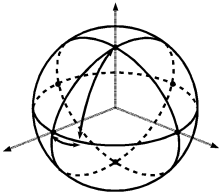My blog has been moved to ariya.ofilabs.com.

## Monday, November 26, 2007

### Fun with rotationsThe following puzzle might be trivial if you study physics and deal with lots of optics. Or, if you are simply good enough with math, especially with geometry.

First, visualize a 3-d Euclidean space where x-y plane is the "floor" and z points "upward". You are somewhere in this space.

Now assume you have two rotation operators denoted as Q and H. The idea is: both have fixed rotation angle but freely chosen rotation axis.

The common thing of both operators is that the rotation axis can be anything but it must lie on the x-y plane (i.e. the floor), so you can have [1 0 0]T or [0 1 0]T as the rotation vector but not [0 0 1]T. This way, the rotation vector can also be specified by its angle with the x axis. If we denote the angles as a and b for the rotation vectors of Q and H, we write the rotation operators as Q(a) and H(b). Hint: the rotation vector of Q(a) is thus [cos(a) sin(a) 0]T.

Next comes the difference. The rotation angle for Q is 90 degrees, while the rotation angle for H is 180 degrees.

Here is the quiz. Supposed you have a point at [0 0 1]T. This point is first rotated by Q(a). The result is then rotated again by H(b). The questions are:

1. What will be the locus of the final result?
(Hint: find the result as function of a and b).

2. Can you replace the whole transformation (for the point at [0 0 1]T only) by just one Q operator? If yes, determine the rotation vector of this Q.
(Hint: compare the answer to first question with the transformation result by only one Q).

Spoiler Warning: if you have passion for puzzles, go away and solve this one by yourself first. Then come back again here and compare it with the following analysis.

Remark

Although at a glance it looks complicated, the questions are quite easy to solve. It is a matter of doing rotation (couldn't be simpler!). The standard 3x3 transformation matrix will do the job, but usually you can do it much faster if you are familiar with quaternion. For convenience, here are the quaternions for Q and H:

Q(a) = 1 + i*cos(a) + j*sin(a)
H(b) = i*cos(b) + j*sin(b)

(Exercise: normalize the above quarternions)

Then, use the quarternions to find the rotation result with the usual quaternion algebra.

Answer to question #1

After rotated by Q(a), the point [0 0 1]T will become [sin(a) -cos(a) 0]. Transform it with H(b) and you will get [-sin(2*b-a) cos(2*b-a) 0]T.

Note: what you get is actually [x y 0]T with:

x = cos(b)*sin(a-b) - sin(b)*cos(a-b)
y = cos(b)*cos(a-b) + sin(b)*sin(a-b)

which could be simplified using some trigonometric relations.

For the trained eyes, [-sin(2*b-a) cos(2*b-a) 0]T is the equation for a circle because sin(2*b-a)^2+cos(2*b-a)^2 = 1. The z coordinate is 0, means the circle is on the floor.

So, the answer to the first question: the locus will be a unit circle on the x-y plane.

Answer to question #2

After rotated by Q(p), the point [0 0 1]T will become [sin(p) -cos(p) 0]T. This is also a unit circle. It means, direct transformation of [0 0 1]T by Q(p) will also give a circle. That's the answer: yes, rotation by Q(a) followed by H(b), for the point at [0 0 1]T, can be replaced by one Q operator.

But how to find p in Q(p)? Recall [-sin(2*b-a) cos(2*b-a) 0]T from the answer to the first question. Comparing it with [sin(p) -cos(p) 0]T yields p = pi - a + 2*b.

Or, you can specify the rotation vector of Q(p) directly as [-cos(2*b-a) -sin(2*b-a) 0]T.

Not too difficult, isn't it?